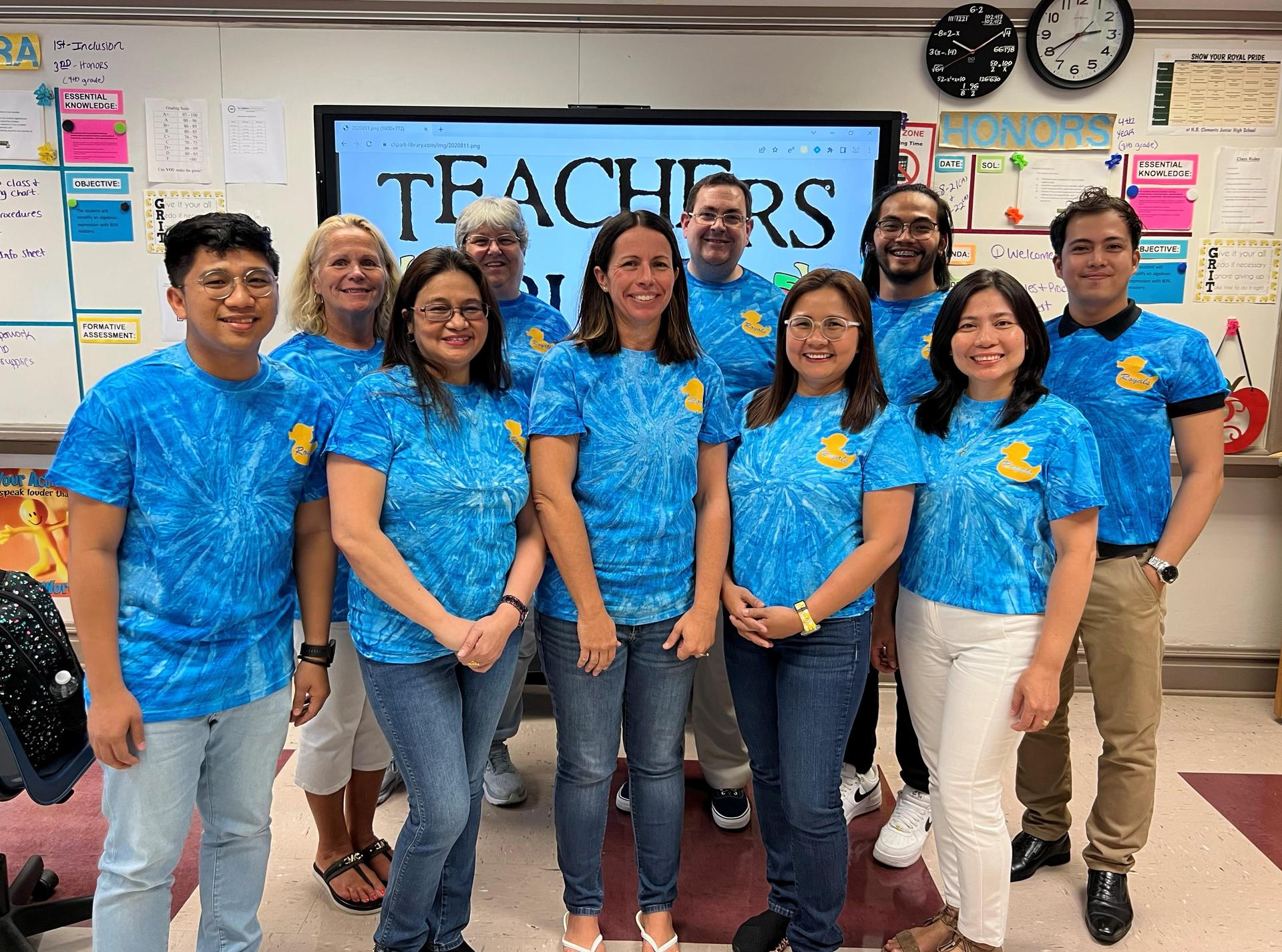# Mathematics

• ### Marsha TsiptsisRow 1:  C. Cordova, D. Pondevida, J. Powers, R. Mamaril, J. Reyes

Row 2:  S. DeGrauwe, M. Tsiptsis, J. Bateson, S. Tolete, W. Elauria

K. Burton

## Math Courses offered to Students at N.B. Clements Junior High School

### Pre-Algebra

This course is designed to prepare students for Algebra I. Students will be required to demonstrate knowledge in all basic mathematical operations and conceptual problem solving. There will be an emphasis on pre-algebra skills such as evaluating and simplifying algebraic expressions and solving multi-step linear equations with variables on both sides. Students will be required to demonstrate proficiency on the SOL tests required by the State Assessment Program.

This course is designed to prepare students for Honors Algebra I. The class covers the same curriculum as Foundations of Algebra, Part III, with differentiation and acceleration of required content. Students must have earned a “C” or better in Advanced Math 7 or a “B” or better in Math 7 AND must have passed the Math 7 SOL. Students will be required to demonstrate proficiency on the SOL tests required by the State Assessment Program.

### Algebra 1 Part 1

This course will explore the same concepts as Algebra I; however, the instruction will be given over a two-year period. This course is designed to meet the needs of the student who needs to understand basic algebraic functions. Upon successful completion of Part 1, students would be expected to enroll in Part 2 the following year. Students must successfully complete both parts in order to meet the state requirements for Algebra I under the Standards of Learning.

### Algebra 1

The properties and operations of the real number system are developed. Other important topics include equations, inequalities, exponents, polynomials, functions, and systems of equations. A central focus of Algebra I is solving linear equations and inequalities, graphing linear functions and solving systems of linear equations. The use of graphing calculators and other technologies are a part of this course. This course has an SOL.

### Honors Algebra 1

Honors Algebra I covers the topics of Algebra I at a more comprehensive level and at an accelerated pace. This provides the opportunity to study topics more in depth and study additional topics. Differentiation of instruction will occur in content, process, and product and is reflected in the nature and scope of student activities and expectations. Independent practice and dependence on previous learning is routine. Advanced skills in mathematics proficiency are absolutely essential to student success. The use of graphing calculators and other appropriate technologies are a part of this course. This course has an SOL.

### Algebra 2

Algebra II provides a thorough treatment of algebraic concepts through the study of functions, polynomials, rational expressions, complex numbers, and systems of equations. A central focus of Algebra II is solving and graphing quadratic equations, rational equations, radical equations, and absolute value equations. Graphing utilities, especially graphing calculators, are essential for the course. College bound students should master this course before leaving high school and preferably before taking the SAT’s. This course has an SOL.

### Honors Algebra 2

Honors Algebra II covers the topics of Algebra II in a more comprehensive level and at an accelerated pace. Also, there is time to cover additional topics important in more advanced mathematics courses. This course is recommended for those planning a career in a field related to math or science. Graphing calculators are essential and are used to facilitate mathematical modeling of many problems in this course. NOTE: Juniors who did not take Geometry until the tenth grade and received an A in Algebra I and Geometry may take Honors Algebra II. This course has an SOL.